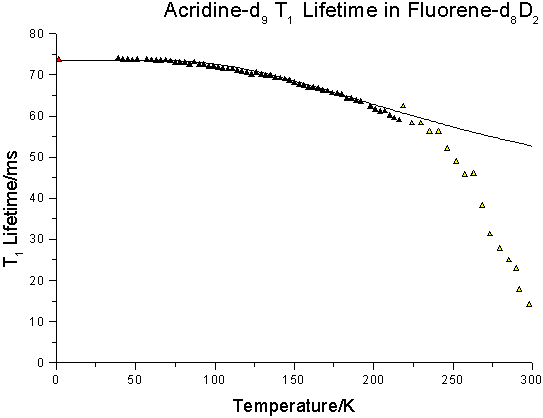The yellow shaded data are from a separate measurement cycle. The red shaded data point at 1.5 K
was obtained by equilibration of the triplet spin sublevel population via microwave irradiation (ODMR).

The solid line in the above figure corresponds to the fit of the function in eq. 1 to the data between 39 and 200 K with.

kTG(T)=13.58 s-1 + 28.75 s-1exp(-501.5 K/T).

As in this temperature region the photoreaction renders only negligible contributions to the triplet decay rate kT(T), the data fit reflects the intramolecular acridine-d9 T1 decay rate in the fluorene-d8D2 host crystal. Such a weak temperature dependence is typical for intramolecular decay rates in organic molecular crystals.

t(T) = 1/kTG(T)=1/(kTG(T=0)+k1exp(-E1/T))                                 (1)

where kTG(T) is the acridine triplet to ground state intramolecular decay rate.

Home Back